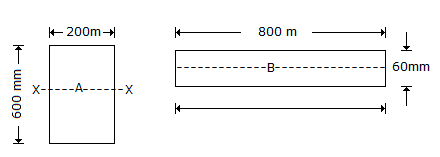# Civil Engineering - UPSC Civil Service Exam Questions

36.

Cross sections of two beams A (600mm x 200mm) and B (800mm x 60mm) are shown in the given figure. Both the beams have the same material. By how many times is the beam A stronger than the beam B in resisting bending ?A. 80 B. 60 C. 50 D. 25

Explanation:

No answer description available for this question. Let us discuss.

37.

Viewing watershed as a system, which one of the following assumptions is made in the unit graph theory ?

 A. Non-linearity B. Both linearity and time variance C. Both time invariance and non-linearity D. Both linearity and time invariance

Explanation:

No answer description available for this question. Let us discuss.

38.

Following statements certain to the position of Sun when it lies over the First Point of Aries :
1. It's hour angle is zero.
2. It's right ascension is zero.
3. It's declination is zero.
Of these statements :

 A. 1 and 3 are correct B. 2 and 3 are correct C. 1 and 2 are correct D. 1, 2 and 3 are correct

Explanation:

No answer description available for this question. Let us discuss.

39.

The bearing capacity factors Nc, Nq and Nr are functions of:

 A. width and depth of footing B. density of soil C. cohesion of soil D. angle of internal friction of soil

Explanation:

No answer description available for this question. Let us discuss.

40.

Budgeting with Bar chart is a tool to find

 A. total cost of project B. total time along with cost of project C. indirect cost of project D. direct cost of project